# Chemistry Science Curriculum Framework

 Page 1/10 Date 23.04.2018 Size 1.01 Mb. #45990

## Revised 2005

Course Title: Chemistry

Course/Unit Credit: 1

Course Number: 421000

Teacher Licensure: Please refer to the Course Code Management System (https://adedata.arkansas.gov/ccms/) for the most current licensure codes.

Chemistry
Chemistry should explore the composition of matter through its properties, its atomic structure, and the manner in which it bonds and reacts with other substances. Students should be expected to use suitable mathematics and collect and analyze data. Instruction and assessment should include both appropriate technology and the safe use of laboratory equipment. Students should be engaged in hands-on laboratory experiences at least 20% of the instructional time.
Strand Standard
 Atomic Theory 1. Students shall understand the historical development of the model of the atom. 2. Student shall understand the structure of the atom. 3. Students shall understand how the arrangement of electrons in atoms relates to the quantum model. Periodicity 4. Students shall understand the significance of the Periodic Table and its historical development. 5. Students shall name and write formulas for binary and ternary compounds. 6. Students shall explain the changes of matter using its physical and chemical properties. 7. Students shall use atomic mass or experimental data to calculate relationships among elements and compounds. Bonding 8. Students shall understand the process of ionic bonding. 9. Students shall understand the process of covalent bonding. 10. Students shall understand the process of metallic bonding. 11. Students shall relate the physical properties as they relate to different types of bonding. Stoichiometry 12. Students shall understand the relationship between balanced chemical equations and mole relationships. 13. Students shall understand the mole concept and Avogadro’s number. 14. Students shall predict the product(s) based upon the type of chemical reaction. 15. Students shall understand the composition of solutions, their formation and their strengths expressed in various units. Gas Laws 16. Students shall understand the behavior of gas particles as it relates to the kinetic theory. 17. Students shall understand the relationship among temperature, pressure, volume and moles of gas. 18. Students shall apply the stoichiometric mass and volume relationships of gases in chemical reactions.

Strand Standard

 Acids and Bases 19. Students shall understand the historical development of the acid/base theories. 20. Students shall apply rules of nomenclature to acids, bases and salts. 21. Students shall understand the general properties of acids, bases and salts. 22. Students shall demonstrate an understanding of titration as a laboratory tool. Kinetics and Energistics 23. Students shall understand enthalpy, entropy, and free energy and their relationship to chemical reactions. Equilibrium 24. Students shall apply rules of nomenclature to acids, bases, and salts. Oxidation-Reduction Reactions 25. Students shall understand oxidation-reduction reactions to develop skills in balancing redox equations. 26. Students shall explain the role of oxidation-reduction reactions in the production of electricity in a voltaic cell. Organic Chemistry 27. Students shall differentiate between aliphatic, cyclic, and aromatic hydrocarbons. 28. Students shall know and describe the functional groups in organic chemistry. 29. Students shall demonstrate an understanding of the role of organic compounds in living and non-living systems. Nuclear Chemistry 30. Students shall understand the process transformations of nuclear radiation. 31. Students shall understand the current and historical ramifications of nuclear energy. Nature of Science 32. Students shall demonstrate an understanding that science is a way of knowing. 33. Students shall design and safely conduct a scientific inquiry. 34. Students shall demonstrate an understanding of current theories in chemistry. 35. Students shall use mathematics, science equipment, and technology as tools to communicate and solve problems in chemistry. 36. Students shall describe the connections between pure and applied science. 37. Students shall describe various careers in chemistry and the training required for the selected career.

Strand: Atomic Theory

Standard 1: Students shall understand the historical development of the model of the atom.

 AT.1.C.1 Summarize the discoveries of the subatomic particles Rutherford’s gold foil Chadwick’s discovery of the neutron Thomson’s cathode ray Millikan’s Oil Drop AT.1.C.2 Explain the historical events that led to the development of the current atomic theory

##### Strand: Atomic Theory

Standard 2: Students shall understand the structure of the atom.
 AT.2.C.1 Analyze an atom’s particle position, arrangement, and charge using: proton neutron electron AT.2.C.2 Compare the magnitude and range of nuclear forces to magnetic forces and gravitational forces AT.2.C.3 Draw and explain nuclear symbols and hyphen notations for isotopes: nuclear symbol: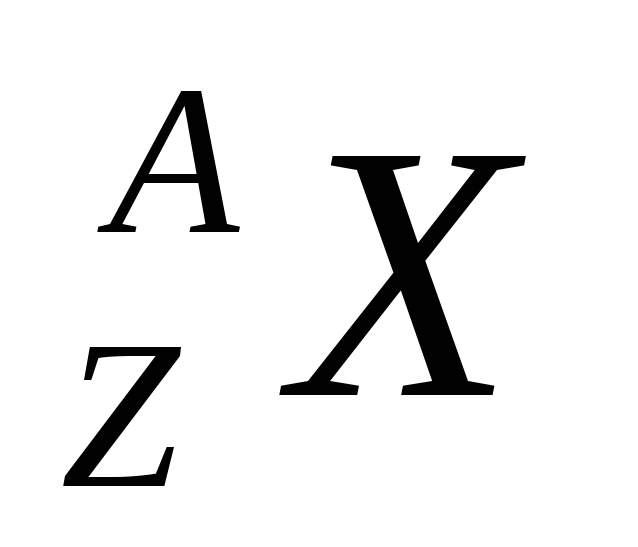Where Hyphen notation: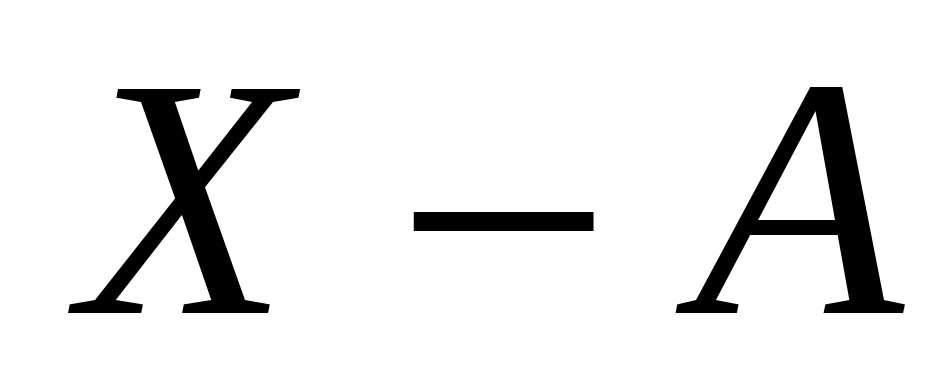Where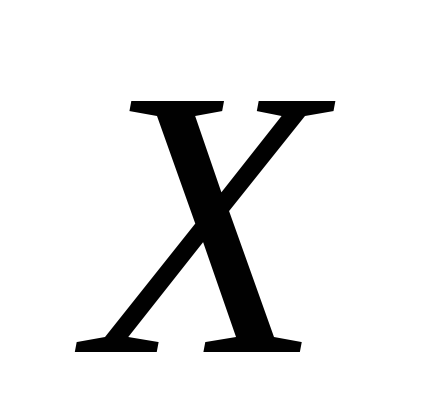= element symbol;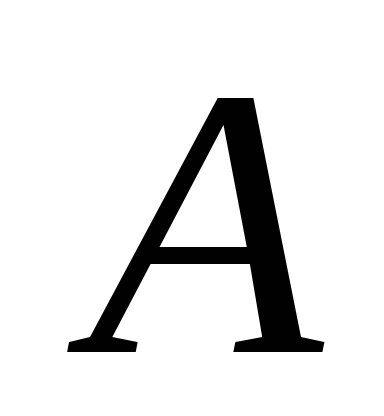= the mass number;= atomic number; the number of neutrons =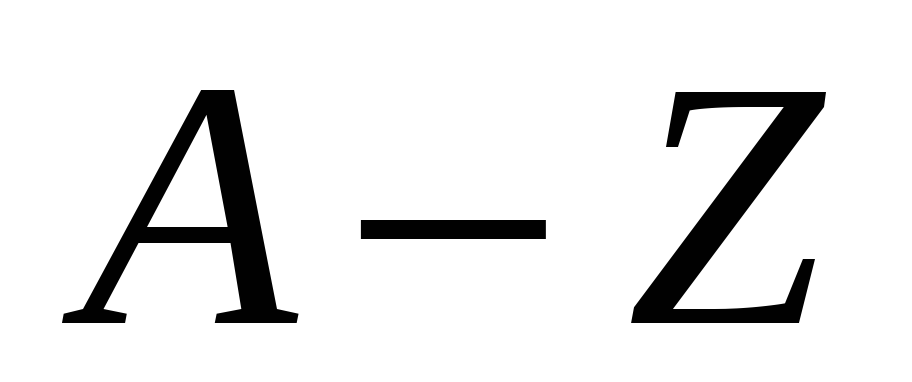AT.2.C.4 Derive an average atomic mass AT.2.C.5 Determine the arrangement of subatomic particles in the ion(s) of an atom